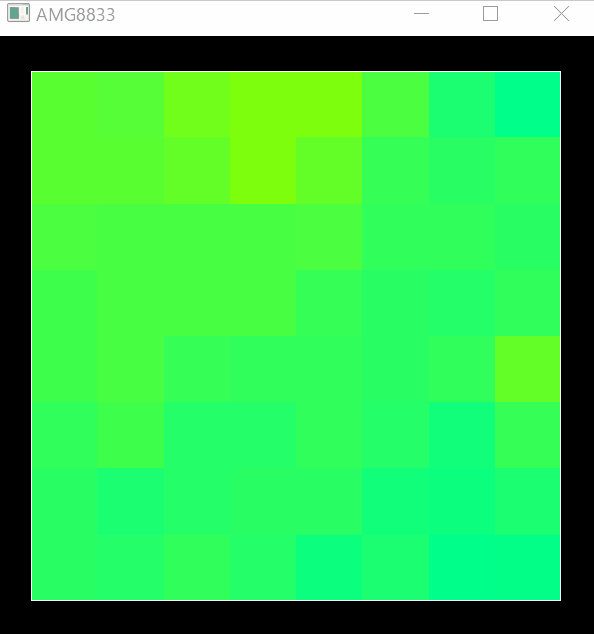# Introduction

This article is a record of using the 8x8 infrared array sensor "AMG8833" from Arduino, sending the data to a PC in real time and visualizing it with Python (PyQt5, PyQtGraph).

It is visualized in real time like the image below.Arduino Arduino acquires thermography data and sends it to a PC (Python) by serial communication. AMG8833 This time, I am using Switch Science's Conta ™ Thermography AMG8833 installed.

According to Switch Science

The temperature measurement range for each element is 0 ° C to 80 ° C. The measurement area is a square pyramid in front of the sensor (about 60 degrees vertically and horizontally), and a two-dimensional image obtained by dividing this area into 8x8 pixels can be obtained.

We also use Conta ™ Base Shield to make AMG8833 easy to use.

## Data acquisition & transmission

There are several AMG8833 libraries listed on the Switch Science page, but here we are using SparkFun's SparkFun_GridEYE_Arduino_Library. The following program is just a slight change of Sample Program.

In serial communication, 8x8 = 64 temperature data are transmitted as character strings separated by ",".

#### `amg8833-serial.ino`

``````
#include <SparkFun_GridEYE_Arduino_Library.h>
#include <Wire.h>

float pixelTable;

GridEYE grideye;

void setup() {
Wire.begin();
//The switch science AMG8833 address is set to 0x68 by default.
grideye.begin(0x68);
Serial.begin(115200);
}

void loop() {
// 8x8=Obtain the temperature for 64 pixels and store it in grideye.
for(unsigned char i = 0; i < 64; i++){
pixelTable[i] = grideye.getPixelTemperature(i);
}
//The temperature of all pixels","Separated by and arranged in one line and transmitted by serial communication.
for(unsigned char i = 0; i < 64; i++){
Serial.print(pixelTable[i]);
if(i != 63){
Serial.print(",");
}
}
Serial.println();
delay(50);
}
``````

Python The heat map is displayed in real time based on the temperature data received from Arduino.

## Library

--pyserial --serial communication

• numpy
• pyqt5 --pyqtgraph --Real-time drawing -(matplotlib) --Color map

Data visualization in Python is matplotlib, but it has the disadvantage that it is too heavy to update the screen in real time with matplotlib. Therefore, instead of matplotlib, we use a lightweight library called pyqtgraph.

However, this time, I am using a part of matpotlib to convert the temperature data to color (RGBA). It seems that pyqtgraph can be similar, but in that case it seems that you need to specify the color to be used for the color map. Here, we use matplotlib, which provides a color map by default.

## Data reception & heat map display

#### `heatmap.py`

``````
from pyqtgraph.Qt import QtGui, QtCore
import matplotlib.cm as cm
import matplotlib as mpl
import pyqtgraph as pg
import numpy as np
import serial

#TEMP to make the heatmap easier to see_MAX=40.Set to 0
TEMP_MIN = 0.0
TEMP_MAX = 40.0

app = QtGui.QApplication([])

win = pg.GraphicsLayoutWidget(show=True, title="AMG8833")
win.resize(600,600)
win.setWindowTitle('AMG8833')
pg.setConfigOptions(antialias=True)

view.setAspectLocked(True)

img = pg.ImageItem(border='w')

#Color Map for converting temperature to color
norm = mpl.colors.Normalize(vmin=TEMP_MIN, vmax=TEMP_MAX)
cmap = cm.jet
m = cm.ScalarMappable(norm=norm, cmap=cmap)

#Serial communication
ser = serial.Serial("COM13", 115200, timeout=1)

#8x8 temperature data
data = np.zeros((8, 8))

def get_data():
global data, ser
if ser.in_waiting:
#Receive data from Arduino
#Exception handling is easy because an error will occur when acquiring data.
try:
temps = [float(t) for t in line.split(",")]
data = np.array(temps).reshape((8, 8))
except:
pass

def update_plot():
global img, data
img.setImage(m.to_rgba(data))

#Data reception every 50ms
timer1 = QtCore.QTimer()
timer1.timeout.connect(get_data)
timer1.setInterval(50)
timer1.start()

#Heatmap update every 100ms
timer2 = QtCore.QTimer()
timer2.timeout.connect(update_plot)
timer2.setInterval(100)
timer2.start()

if __name__ == '__main__':
import sys
if (sys.flags.interactive != 1) or not hasattr(QtCore, 'PYQT_VERSION'):
QtGui.QApplication.instance().exec_()
``````

In the above program, data reception and heatmap update are separated into different QTimers. If you update the heatmap every time you receive data, the heatmap may not be updated in time. To prevent this, data reception and heat map update can be performed separately.